Courses

# Manufacturing Engineering - 2

## 20 Questions MCQ Test GATE Mechanical (ME) 2022 Mock Test Series | Manufacturing Engineering - 2

Description
This mock test of Manufacturing Engineering - 2 for GATE helps you for every GATE entrance exam. This contains 20 Multiple Choice Questions for GATE Manufacturing Engineering - 2 (mcq) to study with solutions a complete question bank. The solved questions answers in this Manufacturing Engineering - 2 quiz give you a good mix of easy questions and tough questions. GATE students definitely take this Manufacturing Engineering - 2 exercise for a better result in the exam. You can find other Manufacturing Engineering - 2 extra questions, long questions & short questions for GATE on EduRev as well by searching above.
*Answer can only contain numeric values
QUESTION: 1

Solution:
QUESTION: 2

### A copper wire is annealed at 250 mm diameter. The smallest diameter to which it could be theoretically drawn in 3 passes is

Solution:

The maximum reduction possible under ideal conditions is given by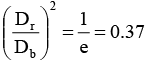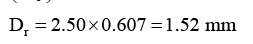In one pass of = 1.52 mm

In second pass of = 1.52 × 0.607 = 0.922 mm

In third pass D i = 0.9222×0.607 = 0.56 mm

QUESTION: 3

### The approximate roll load necessary to reduce 160 cm wide, 0.3 cm thick Al-slat to 0.25 cm thick in one pass, using 30 cm diameter rolls is ( 2 ) 0σ= 9.1 kg / mm

Solution:
QUESTION: 4

The forging load required to transform a 1 m long 1m diameter cylindrical bloom into a square section of equal area in a hydraulic press ( 2 ) 0σ= 450 kg / cm

Solution:
QUESTION: 5

Which one of the following is a continuous bending process in which opposing rolls are used to produce long sections of formed shapes from coil or strip stock?

Solution:
QUESTION: 6

Find the time required on the shaper to complete one cut on a plate 620 mm × 880 mm, if the cutting speed is 6 m/min. the return time to cutting time ratio is 1:5 and the feed is 3 mm/stroke. The clearance at each end is 75 mm.

Solution: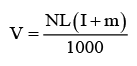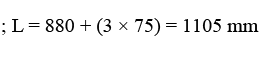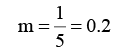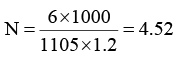Time required,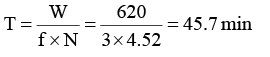QUESTION: 7

The maximum punch force required in punching a 30 mm diameter hole through 3 mm thick annealed aluminum alloy sheet at room temperature is (Take ultimate tensile strength of aluminum alloy as 1000 Mpa)

Solution:
QUESTION: 8

For an orthogonal cutting process on mild steel, assume the following data 12
t = 0.3 mm, t = 0.85 mm, w = 2.5 mm, α= 9°, Ft = 800 N and Ft = 400 N . The ultimate shear stress τs of the work material is (N/mm2)

Solution:

Given t t = 0.3 mm w = 2.5 mm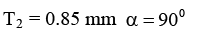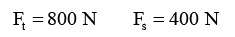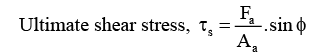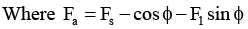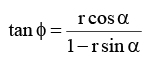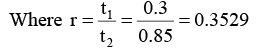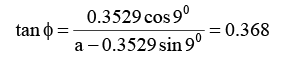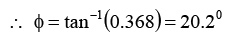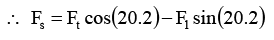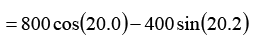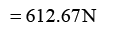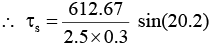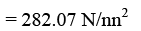QUESTION: 9

Which of the following forces causes power consumption in metal cutting operation?

Solution:
*Answer can only contain numeric values
QUESTION: 10

Find chip reduction co-efficient for the following data; work place material C25 steel, tool signature 0-10-6-7-8-90-1 mm, feed 0.3 mm/rev, depth of cut is 4 mm, chip thickness is 0.48 mm.

Solution:

Chip reduction co-efficient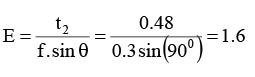QUESTION: 11

A round rod of annealed bras 70-30 is being drawn from a diameter of 8 mm to a diameter of 4 mm at a speed of 0.8 m/s. (k = 895 MPa, n = 0.49)

Q.

The power required in this operation is

Solution:
QUESTION: 12

The percentage change in cutting speed required to give 60% of reduction in tool life (n = 0.3) is

Solution: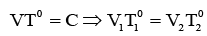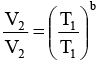There is 60% reduction in the tool life, that means

T2 = 0.4T1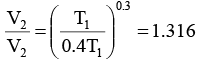V2 should be 31.6% higher than V1 for the required t2 reduciton in tool life.

QUESTION: 13

While machining at 1 m/s, the tool life noted was 120 min. If a similar tool cuts at 25% higher speed, the tool life will be (Take n = 0.32 in the Taylor’s tool life equation)

Solution: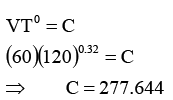Cutting speed in 25% higher i.e., 60 ×1.25= 75 mpm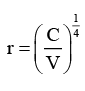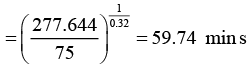QUESTION: 14

The market price of a machine is Rs. 8000 and the discount allowed to distributors is 20% of the market price. For a certain period, it was found that the selling cost was half the factory cost. The material cost, labor cost, and overhead charges of the factory are in the ratio 1:3:2. If the material cost is Rs. 400, then the profit made by the factory on each machine is

Solution:

Market price of machine = 8000/-Discount = 20% or 8000 = Rs. 2000 Selling price of the factor = 8000-2000 = Rs. 6000 Selling price = Factory cost + Admin Cost + Selling Expenses + Profit 4800 = F = 0.5F + P
4800 = 1.5F + P
Factor cost = material cost + Labour cost + Factory overheads = 400 + 1200 + 800 = Rs. 2400 4800 =1.5(2400) + P
P = Rs. 1200

QUESTION: 15

The following part program is used for producing semi circular arc of radius 5 units.
N01 G00 G90 × 5.0 Y5.0 F500 LF
N05 G02 × 5.0 y – 5.0LF

The center of the arc is

Solution:
QUESTION: 16

Crate wear starts at some distance from the tool tip because

Solution:
QUESTION: 17

A 38 mm H.S.S drill is used to drill a hole in a cast iron block 150 mm thick at a cutting speed 30 m/min and feed 0.8 mm/rev. If the over travel of drill is 6 mm and approach 12 mm, the time required to drill the hole is

Solution:
*Answer can only contain numeric values
QUESTION: 18

Consider casing of metal having density 8 × 10-2 N/cc with vertical core having density 2 × 10-2 N/cc and size (all dimensions are in cm) as shown below. The net upward force acting on the core is _________ N.

Solution:
QUESTION: 19

Match List-I with List-II and select the correct answer using the codes given below the lists:

List – I (Moulding)

1. Shell moulding

2. Slush moulding

3. Centrifugal casting

4. Dry sand moulding

List – II (product)

(a) Rain water pipe

(b) Machine tool bed

(c) Cylinder block

(d) Turbine blade

(e) Hollow blade

Solution:
QUESTION: 20

Match List-I with List-II and select the correct answer using the codes given below the lists:

List–I (manufacturing process)

(a) Finish turning

(b) Forming

(c) Thread cutting

(d) Down milling

List–II (Conditions)

1. Back lash eliminator

2. Zero rate

3. Nose radiusing

4. Low speed

Solution: